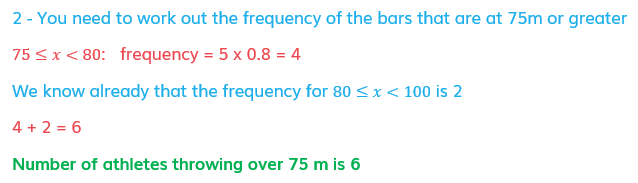# Edexcel IGCSE Maths 复习笔记 6.2.1 Histograms

Edexcel IGCSE Maths 复习笔记 6.2.1 Histograms

#### Aren’t histograms just really hard bar charts?

• No! There are many mathematical differences that you should be aware of but the key difference between a bar chart and a histogram is that with a histogram it is the area of the bars that determine the frequency, on a bar chart it’s the height (or length) of them
• Digging deeper, histograms are used for continuous data (bar charts for discrete) and are particularly useful when data has been grouped into different sized classes
• But the key thing to getting started is that it is the area of the bars that tell us what is happening with the data
• This means, unlike any other graph or chart you have come across, it is very difficult to tell anything from simply looking at a histogram, you have to drill down into the numbers and detail

#### What do I need to know?

• You need to know how to draw a histogram (most questions will get you to finish an incomplete histogram)
• When drawing histograms we will need to use frequency density (fd):• You’ll also need to be able to work backwards from a given histogram to find frequencies and estimate the mean

1. Drawing a histogram

• From a given table you need to work out the frequency density for each class
• Then you can plot the data against frequency density with frequency density on the -axis
• For example, plot a histogram for the following data regarding the average speed travelled by trains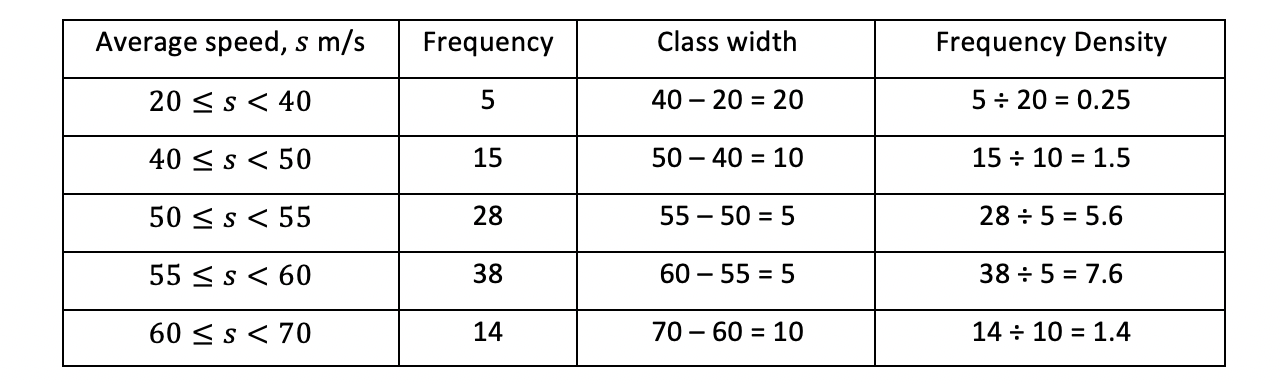• Note that the class width column isn’t essential but it is crucial you show the frequency densities

• Now we draw bars (touching, as the data (speed) is continuous) with widths of the class intervals and heights of the frequency densities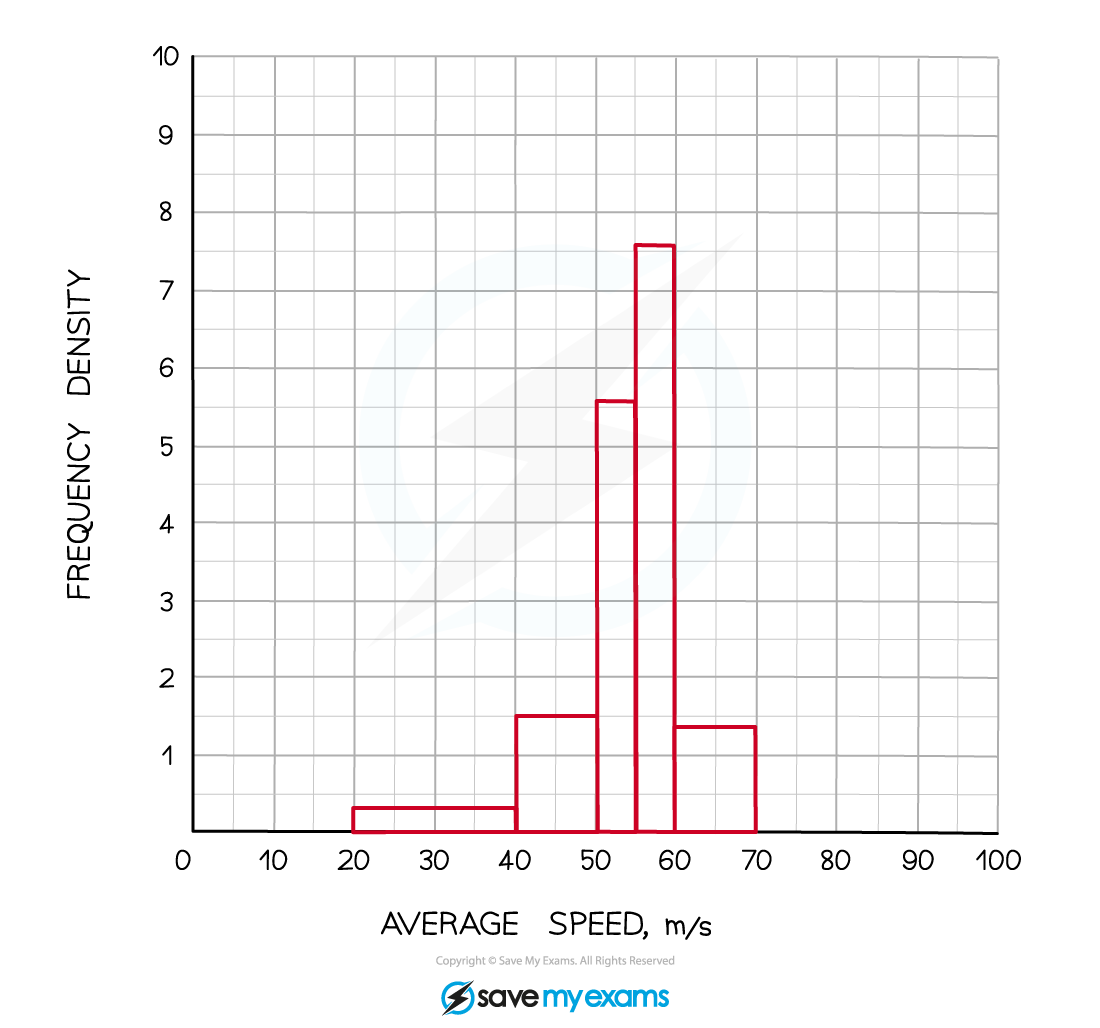2. Interpreting histograms

• We shall still use the example above here but shall pretend we never had the table of data and were only given the finished histogram
• To estimate the mean:
• You need to know the total frequency and what all the data values add up to
• You can’t find the exact total of the data values as this is grouped data but we can estimate it using midpoints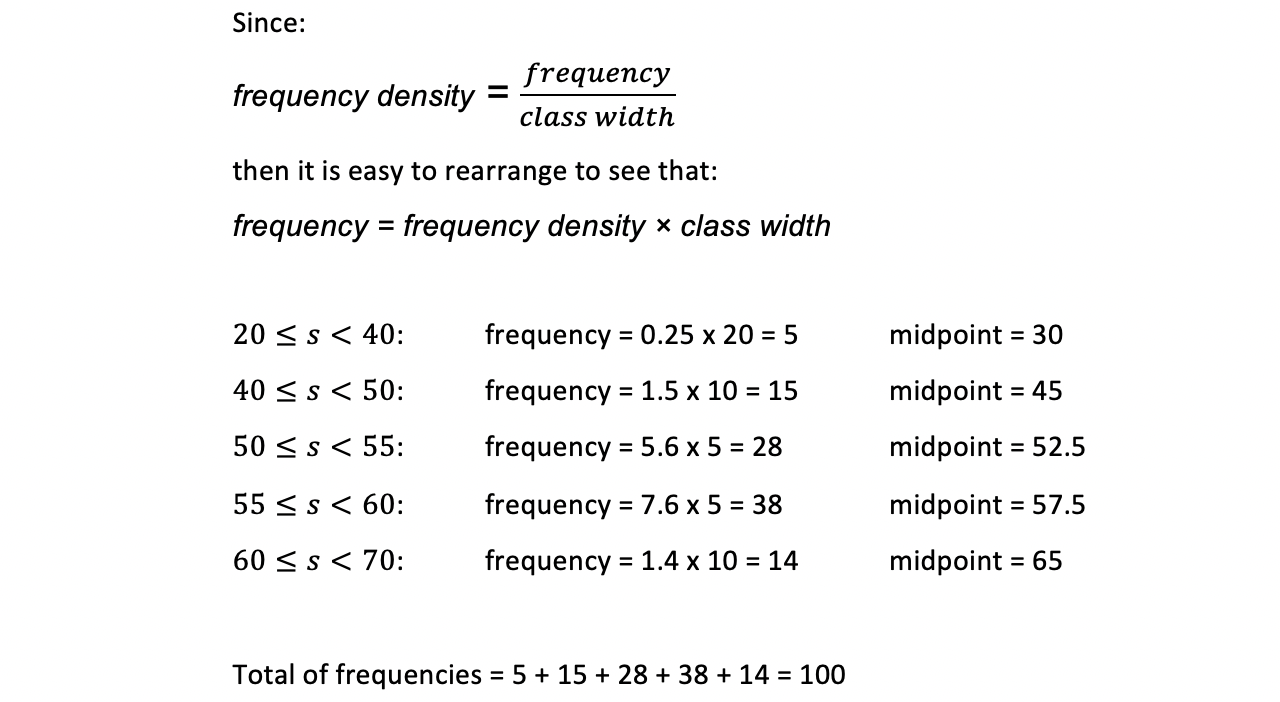• You can draw all of the above in a table if you wish
• Now you can total up (an estimate of) the data values and find the mean:

Total = 5 x 30 + 15 x 45 + 28 x 52.5 + 38 x 57.5 + 14 x 65 = 5390

(Be careful if you type all this into your calculator in one go!)

Estimate of Mean = 5390 ÷ 100 = 53.9

#### Exam Tip

Always work out and write down the frequency densities.  Many students lose marks in exams as they go straight to the graph when asked to draw a histogram and they mess up the calculations. Method marks are available for showing you know to use frequency density rather than frequency.

#### Worked Example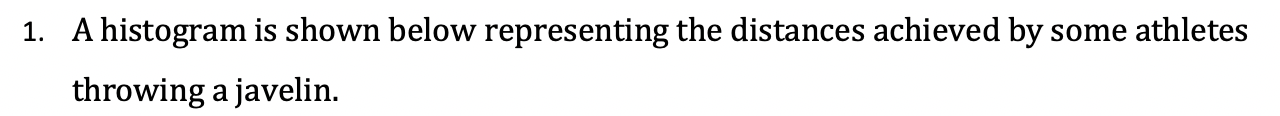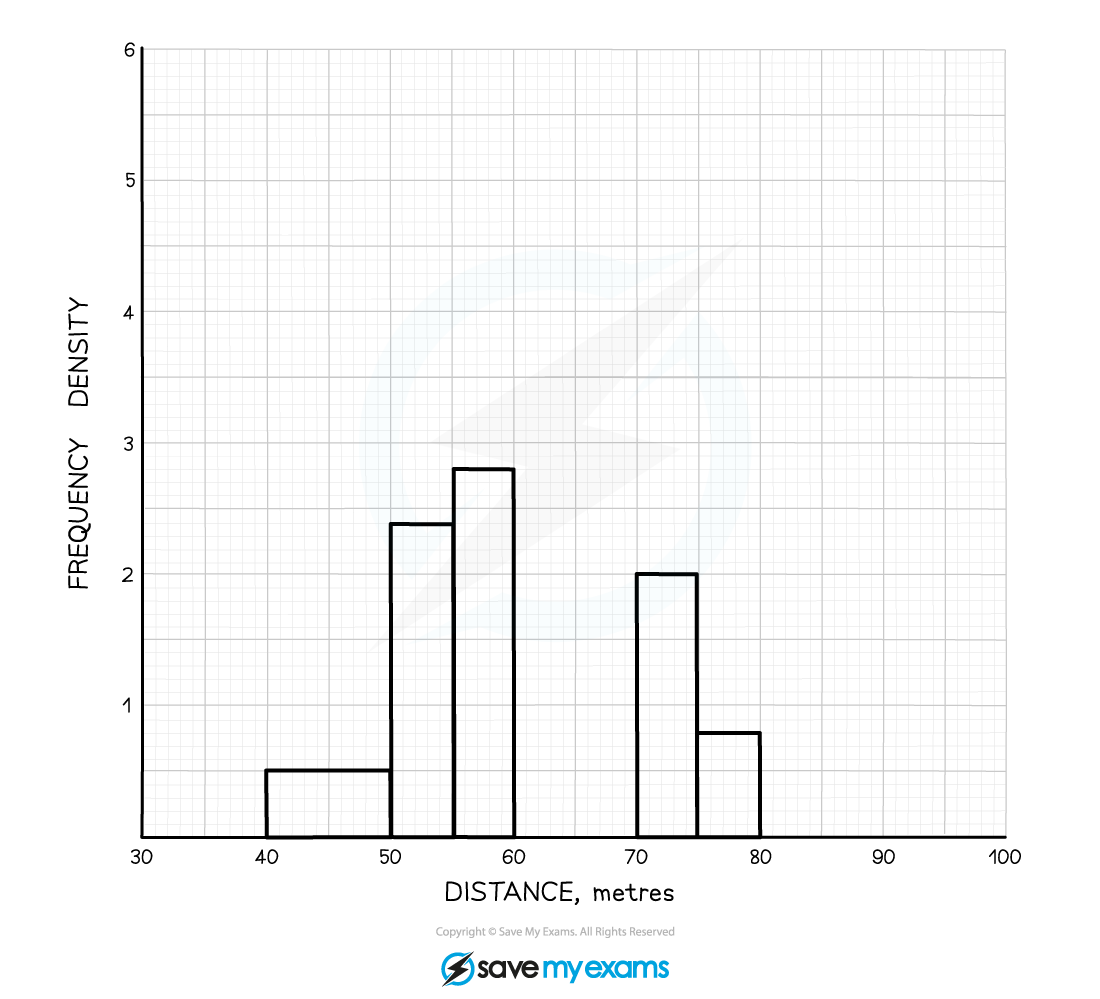#### Worked Example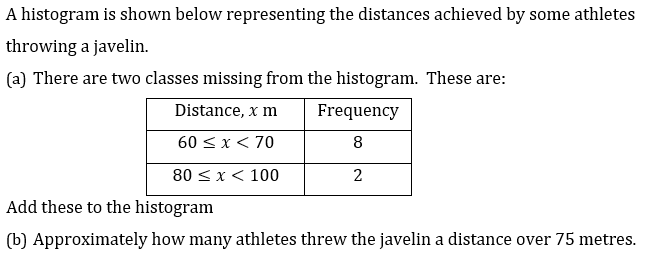#### Worked Example

(a)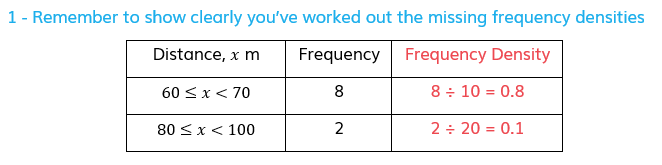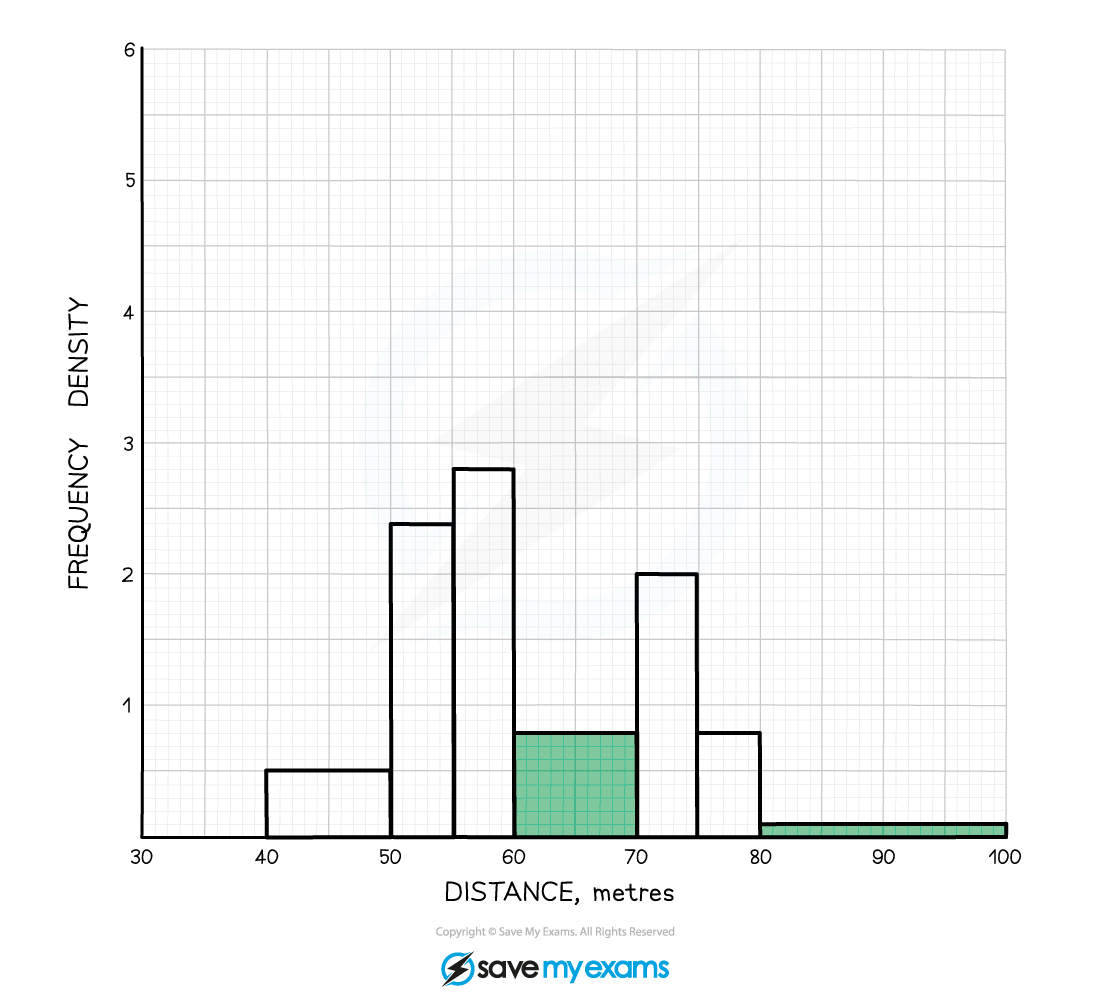#### Worked Example

(b)